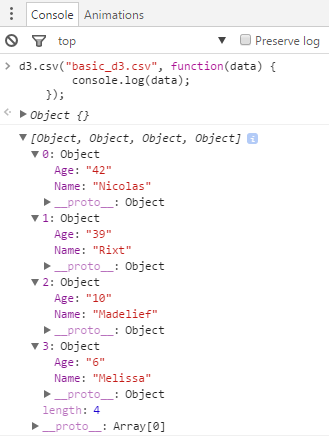# Javascript - Number (Double)

Most programming languages have several types of numeric data, but JavaScript gets away with just one.

All numbers are and are stored as doubles.

## Declaration

``````var myVariable = 10;
var myVariable = +10;
var myVariable = Number(10);```
```

## Max

• 32 bit
````let MAX_INT = 4294967295; // Math.pow(2,32) - 1;`
```
``````Number.MAX_SAFE_INTEGER // 2^53 - 1
Number.MIN_SAFE_INTEGER // (-(2^53 - 1)).```
```
• 64 bit
``````Number.MAX_VALUE; // 2^1024
Number.MIN_VALUE; // 0```
```
• Larger value are represented as Infinity.

## Integer

There is no integer in a javascript.

``````var myNumber = 0.1 + 0.2

console.log("myNumber is not 0.3 but " + myNumber) ```
```

### Coercion

#### Explicit

To convert values to numbers explicitly, you can use:

• the Number function
• or the unary + operator:

Example:

• Adding the + before the string “3” make it a number
````console.log(typeof(+"3"))`
```

#### Implicit

The arithmetic operators -, *, /, and % all attempt to coerce their arguments to numbers before doing their calculation.

The operator + is subtler, because it is overloaded to perform either numeric addition or string concatenation, depending on the types of its arguments.

Silent coercions can also hide errors because it will prevent errors or failure in an arithmetic calculation

#### Value

Variable value Number Value
null 0
true 1
false 0
Non-Number String NaN
Empty String 0
Number String The number represented
Object without the valueOf property NaN
Object with the valueOf property The return value of the valueOf function
Empty Array 0
Non Empty Array with one number value The first number
Non Empty Array with more than one value NaN
undefined NaN
NaN NaN
function NaN

Proof:

``````x = [
null,
true,
false,
"Non-Empty String",
"",
"1",
{ toString: function() { return "Object without valueof"; } },
{ toString: function() { return "Object with valueOf"; } , valueOf: function() { return 10; } },
undefined,
[],
[ 1 ],
[ 1, 2 ],
NaN,
function(){}
];

function numberCoerced(value) {
this.value = value;
this.coercedValue = Number(value);
this.valueType = typeof(value);
}

numbers = [];
for (i in x) {
numbers.push(new numberCoerced(x[i]));
}
console.table(numbers);```
```

### Bitwise

The bitwise arithmetic operators|bitwise arithmetic operators don't operate on their arguments directly as floating-point numbers, they implicitly convert them to 32-bit integers.

## Operators Arithmetic

### Integer and real number

Most operators works on integer and real number (float)

``````function operationArithmetic(desc,opString){
this.descripion = desc;
this.operation = opString;
this.outcome = eval(opString);
}

var ops = [
["Multiplication","0.1 * 1.9"],
["Minus", "21 - 12.3"],
["Division","2.5 / 5"],
["Modulo", "21 % 8"]
];

opsTable = [];
for (i in ops) {
opsTable.push(new operationArithmetic(ops[i],ops[i]));
}
console.table(opsTable);```
```

### String

``````console.log('"10" + 1 ='); // Concat
console.log("10" + 1); // Concat

console.log('1 + "10" = '); // Concat
console.log(1 + "10"); // Concat

console.log('1 + 2 + "10" performs an addition, then a concatenation giving the below result:');
console.log(1 + 2 + "10"); // Addition + Concat

console.log('true + "10" = '); // Concat
console.log(true + "10"); // Concat

console.log('1 + true = ');
console.log(1 + true); // True is coerced to 1```
```
• Minus
``````console.log("10" - 1); // Arithmetic Operation
console.log(10 - "1"); // Arithmetic Operation```
```

## Representation in different base

### Decimal To Binary

• The number 8 in 32-bit integer in binary
``````console.log(    (8).toString(2)    ); // 8 in base 2 (binary)

// 1000
// Exactly 00000000000000000000000000001000 in 32 bit but the 0 at the left are not shown.```
```

where:

• The argument to toString specifies the radix

### Binary to decimal

``````console.log("The number 1001 in base 2 has the representation ("+parseInt("1001", 2 )+") in base 10")
// 1001 is a base 2 (binary) number representation```
```

### Conversion Table (Binary / Hex / Decimal)

``````function numberRepresentation(decimal, binary, hexadecimal) {
this.decimal = decimal;
this.binary = binary;
}
numbers =[]
for (var i=0;i<=16;i++) {
numbers.push(
new numberRepresentation(
i,
i.toString(2),
i.toString(16).toUpperCase()
)
);
}
console.table(numbers);```
```

Recommended PagesComputer - Floating-point (Representation and usage)

Computer representations of floating point numbers typically use a form of rounding to significant figures, but with binary numbers. The number of correct significant figures is closely related to the...D3 - Csv Function (CSV from an URL )

This section talks the function d3.csv that parse a CSV file. To parse a CSV string, see . Reference: Csv The csv file or string must...D3 - DSV

d3-dsv is a library to parse a DSV string (not file) into an array of rows where the property are the headers. To parse a CSV file, see . stringnumbers A template string...
Javascript - Boolean (Truthy|Falsy)

in Javascript. JavaScript defines a list of specific values that are considered “falsy” because when coerced to a boolean, they become false. Any other value not on the “falsy” list is automatically...
Javascript - Integer

Integer aren't implemented in javascript, only double exists. See With integers, you have to take care that all calculations fit within the range between –2^53 and 2^53, but you don’t have to worry...
Javascript - Primitive Data Type

Javascript has 6 primitive data type. string number boolean null undefined symbol Except for null and undefined, all primitive values have object equivalents that wrap around the primitive...
Javascript - Type

Type in Javascript Javascript is not static typed but it exist Javascript wrapper language that implements it. See Only values have types in JavaScript; variables are just simple containers for those...Number - Floating-point (system|notation) - (Float|Double) - Approximate numeric

The term floating point refers to the fact that the number's radix point can float, that is, it can be placed anywhere relative to the significant digits of the number. They are fractional numbers...